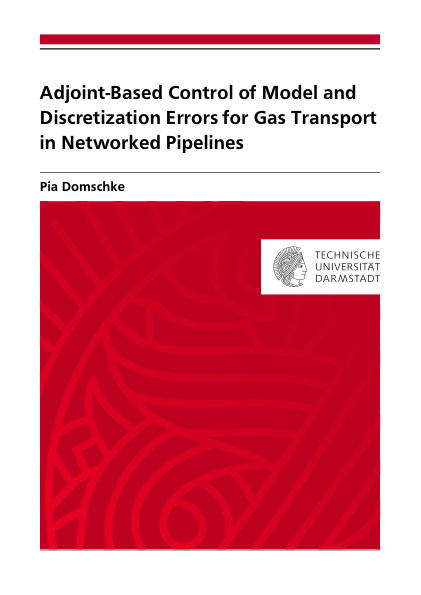Datenbestand vom 20. August 2019aktualisiert am 20. August 2019

# ISBN 9783843900720Euro 60,00 inkl. 7% MwSt

978-3-8439-0072-0, Reihe Mathematik

Pia Domschke
Adjoint-Based Control of Model and Discretization Errors for Gas Transport in Networked Pipelines

131 Seiten, Dissertation Technische Universität Darmstadt (2011), Softcover, A5

## Zusammenfassung / Abstract

We develop an adaptive algorithm to simulate the flow of gas through networks. The aim is to choose the applied models and discretizations in such a way that the computational effort is kept as small as possible while a prescribed accuracy of the solution is maintained.

We first model the gas flow on networks. Those networks mainly consist of pipes but also of compressor stations and valves, which are described by algebraic equations. The models describing the flow of gas through pipelines are based on the Euler equations, a hyperbolic system of partial differential equations. Successive simplifications lead to a hierarchy of models that describe the flow of gas qualitatively different, but also with different computational effort. Simplified models are sufficient in network regions with low activity in the gas transport, while sophisticated models should be used to resolve high dynamics accurately. For the whole network, adequate initial and boundary values as well as coupling conditions at the junctions have to be specified.

To solve the hyperbolic equations on a network, appropriate numerical methods have to be used. First, we introduce the concept of Riemann problems, which are essential to understand the structure of general solutions of hyperbolic problems. Then, we derive finite volume methods, which are suitable to solve those equations since they are conservative and may deal with discontinuities by construction. The use of explicit methods is often restrictive regarding the time step size, since a CFL condition has to be satisfied. Thus, we also present an implicit box scheme, which allows for larger time steps.

Since the network behaviour can change in both space and time, an automatic steering of the model hierarchy as well as the discretization is essential. We want to control the error within a given tolerance and thus need information about the precision of the solution. We derive adjoint equations for the network including initial, coupling and boundary conditions. These equations are suitable to compute gradients for optimization tasks but can also be used to estimate the accuracy of models and the discretization with respect to a given cost functional. With these tools, we develop an algorithm that adaptively controls model and discretization errors in order to maintain a prescribed accuracy of the computed solution. Finally, we present numerical results for two networks and demonstrate the applicability of the algorithm.